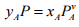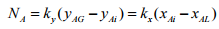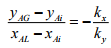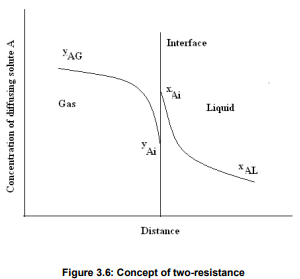Courses

# Interphase Mass Transfer Theory Chemical Engineering Notes | EduRev

## Chemical Engineering : Interphase Mass Transfer Theory Chemical Engineering Notes | EduRev

The document Interphase Mass Transfer Theory Chemical Engineering Notes | EduRev is a part of the Chemical Engineering Course Mass Transfer.
All you need of Chemical Engineering at this link: Chemical Engineering

3.6 INTERPHASE MASS TRANSFER THEORY
The process of mass transfer from the bulk of one phase to the interphse surface and then from the interphase to the bulk of another phase is called interphase mass transfer. As an example absorption of sulfur dioxide from air occurs by water. In this case sulfur dioxide diffuses through air and then passes through the interface between the air and water and finally, diffuses through the adjacent immiscible water phase. Here mass transfer occurs in each phase because of concentration gradient till an equilibrium state (i.e., chemical potential of the component becomes same for both the phases) exists at the interface between the phases. When a system is in equilibrium, there is no net mass transfer between the phases. In cases involving ideal gas and liquid phases, the fairly simple useful relation (Equation (3.71)) known as Raoult’s law can be applied for relating the equilibrium concentrations in the two-phases.(3.71)
where  Pis the vapour pressure of pure solute A at the equilibrium condition and P is the equilibrium pressure. If the liquid phase does not behave ideally, the following modified form of Raoult’s law can be applied:
yAP = xAAPA                                             (3.72)

where YA is the activity coefficient of solute A in solution. For dilute solution Henry’s law can be used to express the equilibrium relations which is expressed by
P= YAP = HxA                                                    (3.73)

where pA is the equilibrium partial pressure of solute A in the vapour phase and H is the Henry’s law constant.

3.6.1 Two-film Resistance Theory
Consider the mass transfer of a solute A from the bulk of a gas phase to the bulk of a liquid phase. This can be shown graphically in terms of distance through the phases as shown in Figure 3.6. The concentration of A in the main body of the gas is yAG mole fraction and it falls to yAi at the interface. In the liquid, the concentration falls from xAi at the interface to xAL in the bulk liquid. According to Lews and Whitman (1924), there is no resistance to solute transfer across the interface separating the phases. Only diffusional resistances are residing in the fluids. The equilibrium concentrations yAi and xAi are obtained from the system’s equilibrium distribution curve. This concept has been called the “two-resistance theory”. In the figure the concentration rise at the interface from yAi to xAi is not a barrier to diffusion in the direction gas to liquid. They are equilibrium concentrations. For steady state mass transfer, the rate at which A reaches the interface from the gas must be equal to the rate at which it diffuses to the bulk liquid, so that no accumulation or depletion of A at the interface occurs. Therefore the mass transfer flux of A in terms of mass transfer film coefficient for each phase can be
written as:(3.74)
After rearranging it can be written as:(3.75)Offer running on EduRev: Apply code STAYHOME200 to get INR 200 off on our premium plan EduRev Infinity!

## Mass Transfer

29 videos|45 docs|44 tests

,

,

,

,

,

,

,

,

,

,

,

,

,

,

,

,

,

,

,

,

,

;